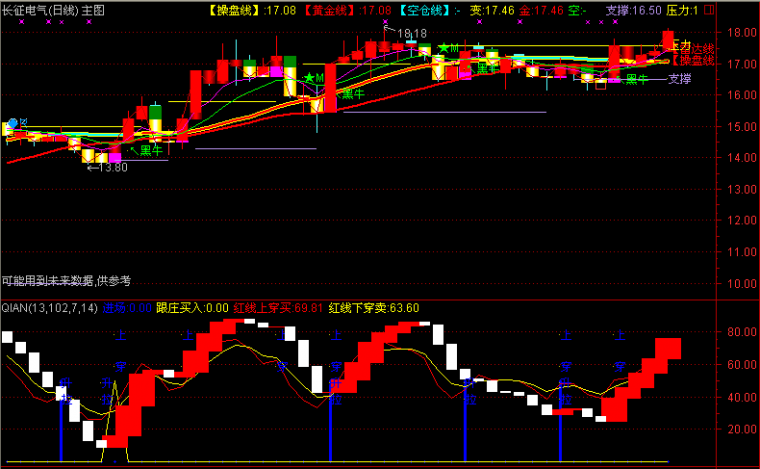﻿ 等待主升选股指标公式公式-通达信公式 －程序化交易（CXH99.COM）

# 等待主升选股指标公式公式[通达信公式]

VA赋值:(2*收盘价+最高价+最低价)/4
VB赋值:5日内最低价的最低值
VC赋值:5日内最高价的最高值
A1赋值:(VA-VB)/(VC-VB)*27的5日指数移动平均
A2赋值:A1的3日指数移动平均
A3赋值:统计3日中满足收盘价的120日简单移动平均-1日前的收盘价的120日简单移动平均的绝对值/收盘价*100<0.2ANDABS(收盘价的250日简单移动平均-1日前的收盘价的250日简单移动平均)/收盘价*100<0.2的天数=3

VA:=(2*C+H+L)/4;VB:=LLV(L,5);VC:=HHV(H,5);A1:=EMA((VA-VB)/(VC-VB)*27,5);A2:=EMA(A1,3);A3:=COUNT(ABS(MA(C,120)-REF(MA(C,120),1))/C*100<0.2 ANDABS(MA(C,250)-REF(MA(C,250),1))/C*100<0.2,3)=3;等待主升:FILTER(WINNER(C)-REF(WINNER(C),1)>0.1 AND A3=1AND MA(C,120)>MA(C,250) AND REF(C,1)/REF(C,10)<1.2 AND C/REF(C,1)<1.05AND CAPITAL<1000000000 AND EVERY(A1>17,3),30) ,LINETHICK3;{程序化交易 www.cxh99.com }(注：由于人数限制，QQ或微信请选择方便的一个联系我们就行，加好友时请简单备注下您的需求，否则无法通过。谢谢您！)

【字体： 】【打印文章】【查看评论

没有相关内容